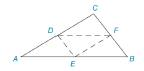Chapter 3.1, Problem 7E### Elementary Geometry for College St...

6th Edition
Daniel C. Alexander + 1 other
ISBN: 9781285195698

#### Solutions

Chapter
Section### Elementary Geometry for College St...

6th Edition
Daniel C. Alexander + 1 other
ISBN: 9781285195698
Textbook Problem
1 views

# In Δ A B C , the midpoints of the sides are joined. (a) what does intuition suggest regarding the relationship between Δ A E D and Δ F D E ? (We will prove this relationship later.) (b) what does intuition suggest regarding Δ A E D and Δ E B F .To determine

a)

To explain:

The intuition regarding the relationship between ΔAED and ΔFDE.

Explanation

Given:

The given figure is shown below.

Approach:

As per given information, the mid-points of the sides are joined in ΔABC. So triangles formed by joining the midpoints will be of equal sides.

Therefore, by SSS postulate, all the four smaller triangles inside ΔABC are congruent

To determine

b)

The intuition regarding the relationship between ΔAED and ΔEBF.

### Still sussing out bartleby?

Check out a sample textbook solution.

See a sample solution

#### The Solution to Your Study Problems

Bartleby provides explanations to thousands of textbook problems written by our experts, many with advanced degrees!

Get Started

#### A sample of n = 9 scores has X = 108. What is the sample mean?

Essentials of Statistics for The Behavioral Sciences (MindTap Course List)

#### Solve the equations in Exercises 126. x1x=0

Finite Mathematics and Applied Calculus (MindTap Course List)

#### True or False: converges mean exists.

Study Guide for Stewart's Multivariable Calculus, 8th

#### What is the goal of a hypothesis test?

Research Methods for the Behavioral Sciences (MindTap Course List)